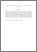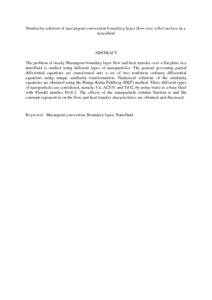# Similarity solution of marangoni convection boundary layer flow over a flat surface in a nanofluid

## Citation

Md. Arifin, Norihan and Nazar, Roslinda and Pop, Ioan (2013) Similarity solution of marangoni convection boundary layer flow over a flat surface in a nanofluid. Journal of Applied Mathematics, 2013. art. no. 634746. pp. 1-8. ISSN 1110-757X

## Abstract

The problem of steady Marangoni boundary layer flow and heat transfer over a flat plate in a nanofluid is studied using different types of nanoparticles. The general governing partial differential equations are transformed into a set of two nonlinear ordinary differential equations using unique similarity transformation. Numerical solutions of the similarity equations are obtained using the Runge-Kutta-Fehlberg (RKF) method. Three different types of nanoparticles are considered, namely, Cu, Al2O3, and TiO2, by using water as a base fluid with Prandtl number Pr=6.2. The effects of the nanoparticle volume fraction φ and the constant exponent m on the flow and heat transfer characteristics are obtained and discussed.Preview
PDF (Abstract)
Similarity solution of marangoni convection boundary layer flow over a flat surface in a nanofluid.pdfView Item